Courses

# RD Sharma Solutions Ex-13.3, (Part -2), Linear Equation In Two Variables, Class 9, Maths Class 9 Notes | EduRev

## Class 9 : RD Sharma Solutions Ex-13.3, (Part -2), Linear Equation In Two Variables, Class 9, Maths Class 9 Notes | EduRev

The document RD Sharma Solutions Ex-13.3, (Part -2), Linear Equation In Two Variables, Class 9, Maths Class 9 Notes | EduRev is a part of the Class 9 Course RD Sharma Solutions for Class 9 Mathematics.
All you need of Class 9 at this link: Class 9

Q8: From the choices given below, choose the equations whose graph is given in fig

(i) y = x
(ii) x + y = 0
(iii) y = 2x
(iv) 2 + 3y = 7x

Ans: We are given co-ordinates (1, – 1) and (-1, 1) as the solution of one of the following equations.

We will substitute the value of both co-ordinates in each of the equation and find the equation which satisfies the given co-ordinates.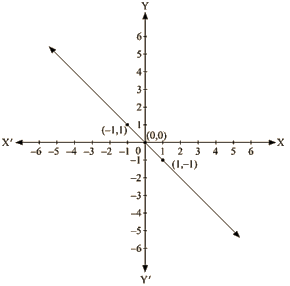(i) We are given, y = x

Substituting x =I and y = -1 ,

we get; 1 ≠ -1

L.H.S ≠ R.H.S

Substituting x = -1  and y = 1 ,

we get; -1 ≠1

L.H.S ≠R.H.S

Therefore, the given equation y = x does not represent the graph in the figure.

(ii) We are given,

x + y = 0

Substituting x =1 and y = -1 , we get

⇒1 + (-1) = 0

⇒ 0 = 0

L.H.S = R.H.S

Substituting x = —1 and y = 1 ,we get

(-1)+ 1 = 0

0 = 0

L.H.S = R.H.S

Therefore, the given solutions satisfy this equation.

Thus, it is the equation whose graph is given.

Q9: From the choices given below, choose the equation whose graph is given fig:

(i) y = x + 2
(ii) y = x – 2
(iii)y = – x + 2
(iv) x + 2y = 6

Ans. We are given co-ordinates (-1, 3) and (2, 0) as the solution of one of the following equations.

We will substitute the value of both co-ordinates in each of the equation and find the equation which satisfies the given co-ordinates.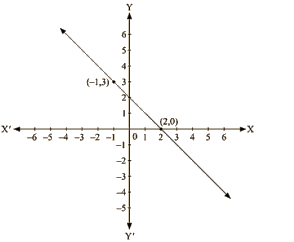(i) We are given, y = x+2

Substituting x = – 1 and y = 3 ,we get

3 ≠ – 1 + 2

L.H.S ≠ R.H.S

Substituting x = 2 and y = 0 ,we get

0 ≠ 4

L.H.S ≠R.H.S

Therefore, the given solution does not satisfy this equation.

(ii) We are given, y = x – 2

Substituting x = —1 and y = 3 ,we get

3 = – 1 – 2

L.H.S ≠ R.H.S

Substituting x = 2 and y = 0 ,we get

0 = 0

L.H.S = R.H.S

Therefore, the given solutions does not completely satisfy this equation.

(iii) We are given, y = – x + 2

Substituting x = – 1 and y = 3,we get

3 = – (– 1) + 2

L.H.S = R.H.S

Substituting x = 2 and y = 0 ,we get

0 = -2 + 2

0 = 0

L.H.S = R.H.S

Therefore, the given solutions satisfy this equation.

Thus, it is the equation whose graph is given.

Q 10 : If the point (2, -2) lies on the graph of linear equation, 5x + 4y = 4, find the value of k.

Ans. It is given that the point (2,-2) lies on the given equation,

5x + ky = 4

Clearly, the given point is the solution of the given equation.

Now, Substituting x = 2 and y = – 2 in the given equation, we get 5x + ky = 4

5 x 2 + (– 2) k = 4

2k = 10 – 4

2k = 6

k = 6/2

k = 3

Q 11 : Draw the graph of equation 2x + 3y = 12. From the graph, find the co ordinates of the point:

(i) whose y-coordinate is 3
(ii) whose x coordinate is -3

Ans. We are given,

2x +3y =12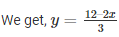Substituting, x = 0 in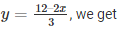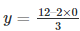y = 4

Substituting x = 6 in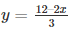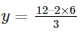y = 0

Thus, we have the following table exhibiting the abscissa and ordinates of points on the line represented by the given equation

 X 0 6 Y 4 0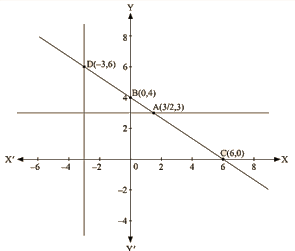By plotting the given equation on the graph, we get the point B (0, 4) and C (6,0).

(i) Co-ordinates of the point whose y axis is 3 are A (3/2, 3)

(ii) Co-ordinates of the point whose x -coordinate is —3 are D (-3, 6)

Q 12: Draw the graph of each of the equations given below. Also, find the coordinates of the points where the graph cuts the coordinate axes:

(i) 6x – 3y = 12
(ii) – x + 4y = 8
(iii) 2x + y = 6
(iv) 3x + 2y + 6 = 0

Ans. (i) We are given,

6x – 3y = 12 We get,

y = (6x —12) /3

Now, substituting x = 0 in y = – (6x – 12)/3 we get

y =- 4

Substituting x = 2 in y = (- 6x —12)/3, we get

y = 0

Thus, we have the following table exhibiting the abscissa and ordinates of points on the line represented by the given equation

 x 0 2 y -4 0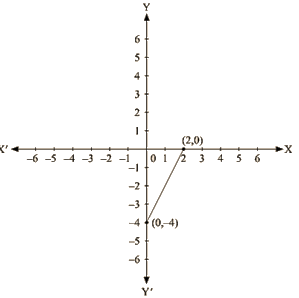Co-ordinates of the points where graph cuts the co-ordinate axes are y = – 4 at y axis and x = 2 at x axis. (ii) We are given,

– x + 4y = 8

We get,

Now, substituting x = 0 in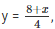we get

y = 2

Substituting x = -8 inwe get

y = 0

Thus, we have the following table exhibiting the abscissa and ordinates of points on the line represented by the given equation

 X 0 -8 Y 2 0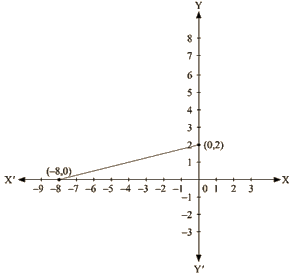Co-ordinates of the points where graph cuts the co-ordinate axes are y = 2 at y axis and x = —8 at x axis.

(iii) We are given,

2x + y = 6

We get, y = 6 – 2x

Now, substituting x = 0 in y = 6 -2x we get

y = 6

Substituting x = 3 in y = 6-2x, we get

y = 0

Thus, we have the following table exhibiting the abscissa and ordinates of points on the line represented by the given equation

 X 0 3 Y 6 0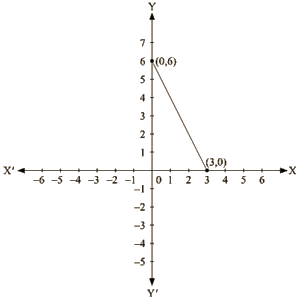Co-ordinates of the points where graph cuts the co-ordinate axes are y = 6 at y axis and x =3 at x axis.

(iv) We are given,

3x+2y+6 = 0

We get,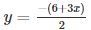Now, substituting x = 0 in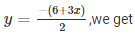y= – 3

Substituting x = —2 in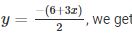y = 0

Thus, we have the following table exhibiting the abscissa and ordinates of points on the line represented by the given equation

 X 0 -2 y -3 0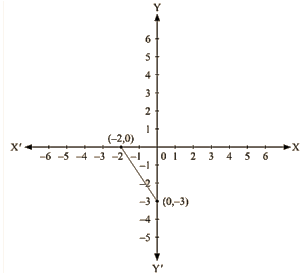Co-ordinates of the points where graph cuts the co-ordinate axes are y = – 3 at y axis and x = – 2 at x axis.

Q 13 : Draw the graph of the equation 2x + y = 6. Shade the region bounded by the graph and the coordinate axes. Also, find the area of the shaded region.

Ans. We are given,

2x + y = 6

We get,

y = 6 – 2x

Now, substituting x = 0 in y = 6 – 2x,

we get y = 6

Substituting x =3 in y = 6— 2x,

we get y = 0

Thus, we have the following table exhibiting the abscissa and ordinates of points on the line represented by the given equation

 X 0 3 Y 6 0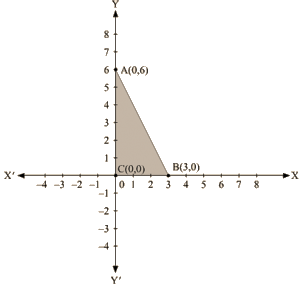The region bounded by the graph is ABC which forms a triangle.

AC at y axis is the base of triangle having AC = 6 units on y axis.

BC at x axis is the height of triangle having BC = 3 units on x axis.

Therefore, Area of triangle ABC, say A is given by A = (Base x Height)/2

A = (AC x BC)/2

A = (6 x 3)/2

A = 9 sq. units

Q 14 : Draw the graph of the equation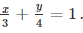Also, find the area of the triangle formed by 3 4 the line and the coordinates axes.

Ans. We are given.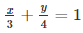4x +3y = 12

We get,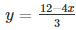Now, substituting x = 0 in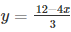,we get

y = 4

Substituting x = 3 in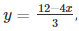we get

y = 0

Thus, we have the following table exhibiting the abscissa and ordinates of points on the line represented by the given equation

 X 0 3 Y 4 0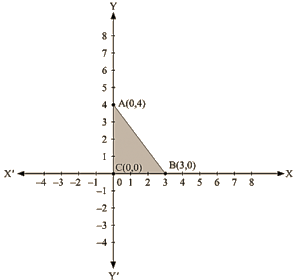The region bounded by the graph is ABC which forms a triangle.

AC at y axis is the base of triangle having AC = 4 units on y axis.

BC at x axis is the height of triangle having BC = 3 units on x axis.

Therefore,

Area of triangle ABC, say A is given by

A = (Base x Height)/2

A= (AC x BC)/2

A = (4 x 3)/2

A = 6 sq. units

Offer running on EduRev: Apply code STAYHOME200 to get INR 200 off on our premium plan EduRev Infinity!

91 docs

,

,

,

,

,

,

,

,

,

,

,

,

,

,

,

,

,

,

,

,

,

,

,

,

,

,

,

,

,

,

,

,

,

;